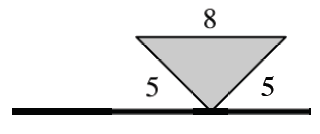### Home > APCALC > Chapter 4 > Lesson 4.3.2 > Problem4-124

4-124.

A horizontal flag is shown below.1. Imagine rotating the flag about its pole and describe the resulting three-dimensional figure. Draw a picture of this figure on your paper.

Imagine a cylinder with cones carved out of the top and bottom.

2. Calculate the volume of the rotated flag.

Prism: $V = Bh$
Cylinder: $V = πr^{2}h$
Sphere: $V=\frac{4}{3}\pi r^3$
Pyramid: $V=\frac{1}{3}Bh$
Cone: $V=\frac{1}{3}\pi r^2h$

$\text{Volume = cylinder }-2(\text{cone})=\pi r^{2}h-2\left ( \frac{1}{3}\pi r^{2}h \right )$

$48\pi\text{ units}^{3}$

Use the eTool below to visualize the flag.
Click the link at right for the full version of the eTool: Calc 4-124 HW eTool